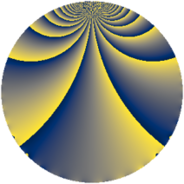# Properties

 Label 760.2.kLevel $760$ Weight $2$ Character orbit 760.k Rep. character $\chi_{760}(229,\cdot)$ Character field $\Q$ Dimension $108$ Newform subspaces $1$ Sturm bound $240$ Trace bound $0$

# Related objects

## Defining parameters

 Level: $$N$$ $$=$$ $$760 = 2^{3} \cdot 5 \cdot 19$$ Weight: $$k$$ $$=$$ $$2$$ Character orbit: $$[\chi]$$ $$=$$ 760.k (of order $$2$$ and degree $$1$$) Character conductor: $$\operatorname{cond}(\chi)$$ $$=$$ $$40$$ Character field: $$\Q$$ Newform subspaces: $$1$$ Sturm bound: $$240$$ Trace bound: $$0$$

## Dimensions

The following table gives the dimensions of various subspaces of $$M_{2}(760, [\chi])$$.

Total New Old
Modular forms 124 108 16
Cusp forms 116 108 8
Eisenstein series 8 0 8

## Trace form

 $$108q + 108q^{9} + O(q^{10})$$ $$108q + 108q^{9} - 8q^{10} - 8q^{14} + 8q^{16} - 8q^{24} + 4q^{25} + 44q^{30} + 40q^{34} - 48q^{36} - 32q^{39} - 4q^{40} - 8q^{41} + 24q^{44} + 16q^{46} - 108q^{49} - 64q^{50} - 72q^{54} - 32q^{55} + 24q^{56} - 4q^{60} - 24q^{64} - 24q^{65} - 64q^{66} + 36q^{70} + 32q^{71} + 48q^{74} + 28q^{80} + 92q^{81} + 16q^{84} - 56q^{86} - 56q^{89} - 28q^{90} - 64q^{94} + 16q^{96} + O(q^{100})$$

## Decomposition of $$S_{2}^{\mathrm{new}}(760, [\chi])$$ into newform subspaces

Label Dim. $$A$$ Field CM Traces $q$-expansion
$$a_2$$ $$a_3$$ $$a_5$$ $$a_7$$
760.2.k.a $$108$$ $$6.069$$ None $$0$$ $$0$$ $$0$$ $$0$$

## Decomposition of $$S_{2}^{\mathrm{old}}(760, [\chi])$$ into lower level spaces

$$S_{2}^{\mathrm{old}}(760, [\chi]) \cong$$ $$S_{2}^{\mathrm{new}}(40, [\chi])$$$$^{\oplus 2}$$